• import torch import torch.nn as nn ... """ 点积注意力机制""" def __init__(self, attention_dropout=0.0): super(dot_attention, self).__init__() self.dropout = nn.Dropout(attention_dropout)
import torch
import torch.nn as nn
import numpy as np

class dot_attention(nn.Module):
""" 点积注意力机制"""

def __init__(self, attention_dropout=0.0):
super(dot_attention, self).__init__()
self.dropout = nn.Dropout(attention_dropout)
self.softmax = nn.Softmax(dim=2)

def forward(self, q, k, v, scale=None, attn_mask=None):
"""
前向传播
:param q:
:param k:
:param v:
:param scale:
:return: 上下文张量和attention张量。
"""
attention = torch.bmm(q, k.transpose(1, 2))
if scale:
attention = attention * scale        # 是否设置缩放
# 计算softmax
attention = self.softmax(attention)
# 添加dropout
attention = self.dropout(attention)
# 和v做点积。
context = torch.bmm(attention, v)
return context, attention

if __name__ == '__main__':
q = torch.ones((1, 2, 512))
k = torch.ones((1, 17, 512))
v = k
attention = dot_attention()
context, attention = attention(q, k, v)
print("context:", context.size(), context)
print("attention:", attention)

展开全文自然语言处理
• ## PyTorch 对应点相乘、矩阵相乘

万次阅读 多人点赞 2018-09-07 21:14:27
一，对应相乘，x.mul(y) ，即点乘操作，点乘不求和操作，又可以叫作Hadamard product；点乘再求和，即为卷积 data = [[1,2], [3,4], [5, 6]] tensor = torch.FloatTensor(data) tensor Out: tensor([[ 1.,...
一，对应点相乘，x.mul(y) ，即点乘操作，点乘不求和操作，又可以叫作Hadamard product；点乘再求和，即为卷积
>>> a = torch.Tensor([[1,2], [3,4], [5, 6]])
>>> a
tensor([[1., 2.],
[3., 4.],
[5., 6.]])
>>> a.mul(a)
tensor([[ 1.,  4.],
[ 9., 16.],
[25., 36.]])

# a*a等价于a.mul(a)

二，矩阵相乘，x.mm(y) ， 矩阵大小需满足： (i, n)x(n, j)
>>> a
tensor([[1., 2.],
[3., 4.],
[5., 6.]])
>>> b = a.t()  # 转置
>>> b
tensor([[1., 3., 5.],
[2., 4., 6.]])

>>> a.mm(b)
tensor([[ 5., 11., 17.],
[11., 25., 39.],
[17., 39., 61.]])


展开全文• PyTorch知识总结前言Numpy1.np.random模块常用函数2.Numpy数组创建函数3.Numpy算术运算4.Numpy中改变向量形状的函数5.合并数组6.Numpy中的几个常用通用函数7.Numpy广播机制PyTorch概述1.Pytorch主要包组成：2....


Numpy & PyTorch知识点总结
前言Numpy1.np.random模块常用函数2.Numpy数组创建函数3.Numpy算术运算4.Numpy中改变向量形状的函数5.合并数组6.Numpy中的几个常用通用函数7.Numpy广播机制
PyTorch概述1.Pytorch主要包组成：2.PyTorch与Numpy区别

1.创建Tensor2.修改Tensor的形状3.常用选择操作函数4.常见逐元素操作5.常见归并操作6.常见比较操作7.常用矩阵函数8.PyTorch与Numpy函数对照表

前言
最近在学习PyTorch，发现PyTorch的tensor与Numpy有不少的相似点，于是重新系统的复习了一下Numpy，并在记录想两者的一些知识点，以备不时之需。
Numpy
1.np.random模块常用函数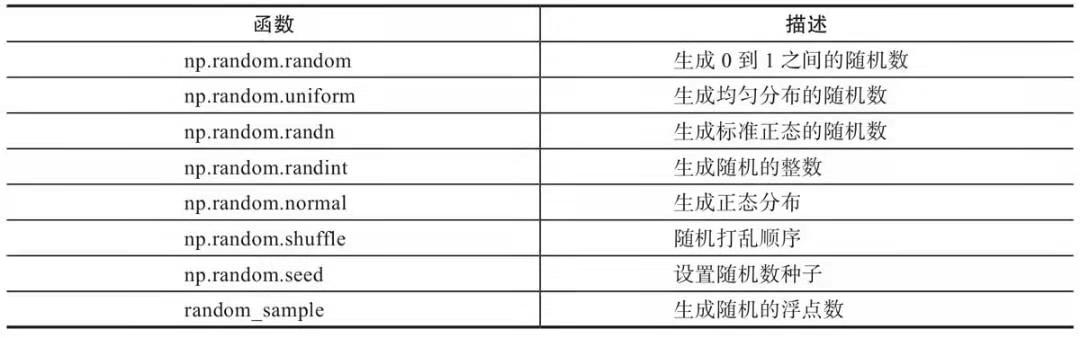np.random.random([3, 3]) #生成3行3列0到1之间随机数
np.random.randn(2, 3)    #生成2行3列标准正态分布随机数

2.Numpy数组创建函数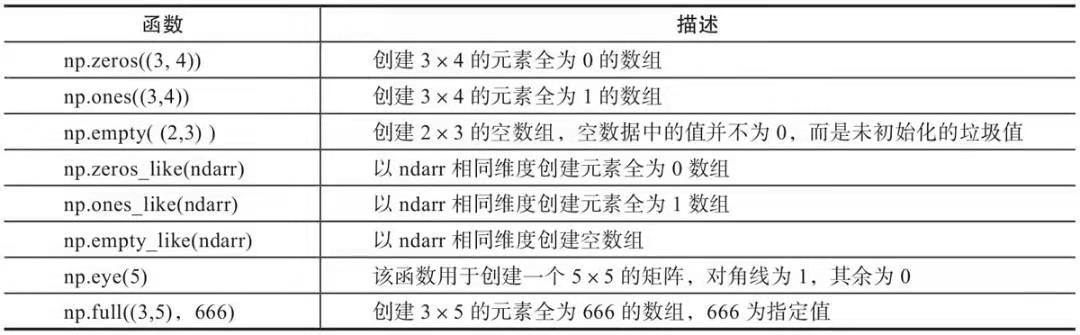#补充
np.arrange([start,] stop[,step],dtype=None)
np.linspace(start, stop, num=50,endpoint=True, retstep=False, dtype=None) #自动生成线性等分向量

3.Numpy算术运算
1.np.multiplay：数组或矩阵对应元素相乘，输出与相乘数组或矩阵大小一致。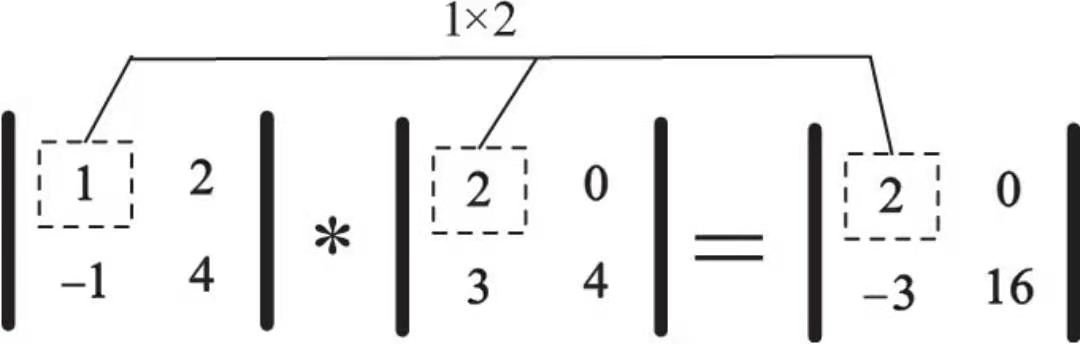2.np.dot()：內积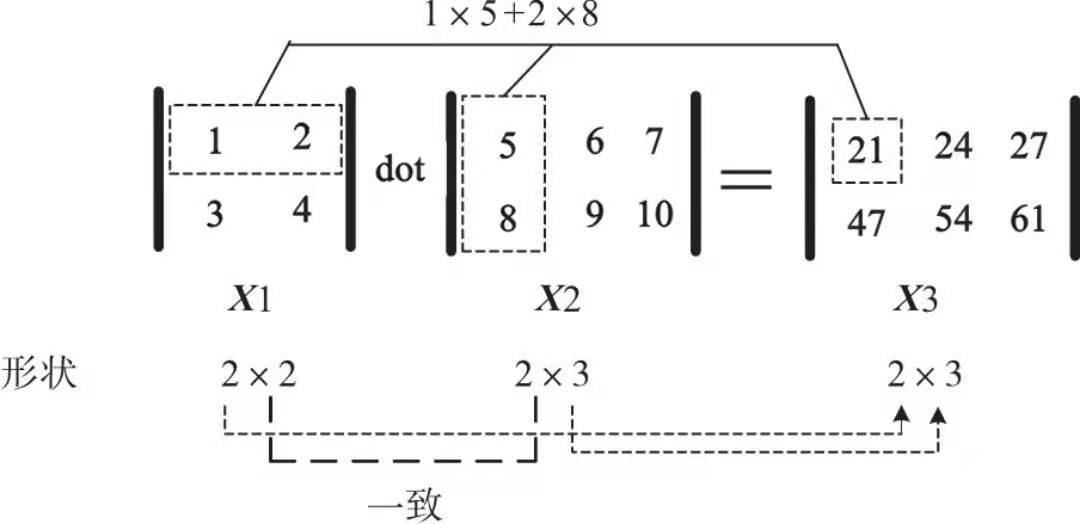4.Numpy中改变向量形状的函数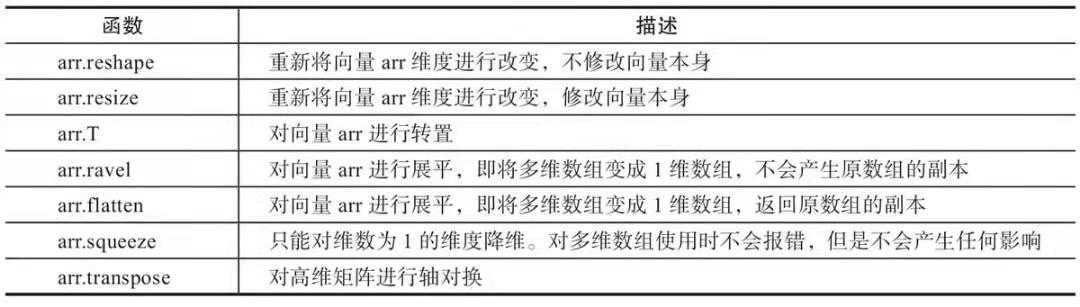补充：transpose在深度学习中常用将图片中表示颜色顺序的RGB改为GBR
5.合并数组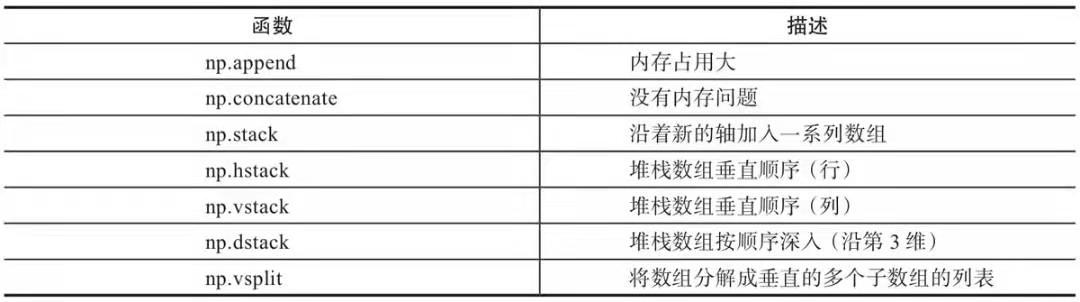6.Numpy中的几个常用通用函数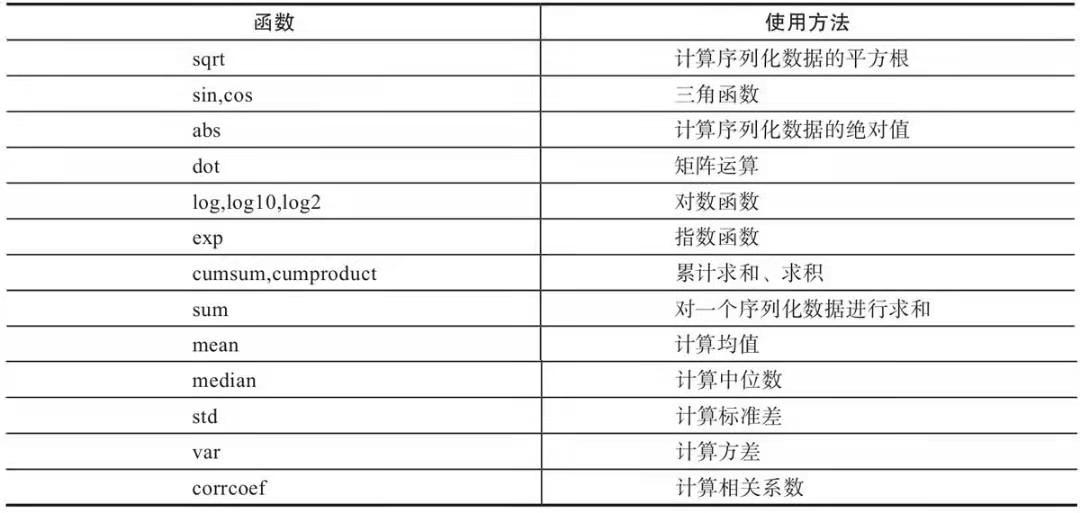（PS：numpy函数比math函数速度更快）
7.Numpy广播机制
当数组的shape不相等时，Numpy会使用广播机制。广播规则：
1）让所有输入数组都向其中shape最长的数组看齐，不足的部分则通过在前面加1补齐，如：
a：2×3×2
b：3×2
则b向a看齐，在b的前面加1，变为：1×3×2
2）输出数组的shape是输入数组shape的各个轴上的最大值；
3）如果输入数组的某个轴和输出数组的对应轴的长度相同或者某个轴的长度为1时，这个数组能被用来计算，否则出错；
4）当输入数组的某个轴的长度为1时，沿着此轴运算时都用（或复制）此轴上的第一组值。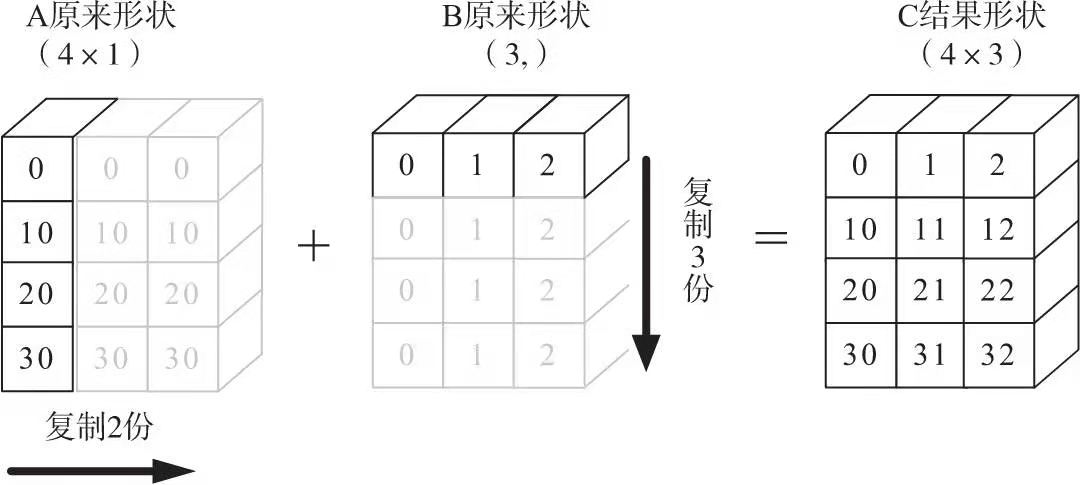PyTorch
概述
1.Pytorch主要包组成：
2.PyTorch与Numpy区别
1.创建Tensor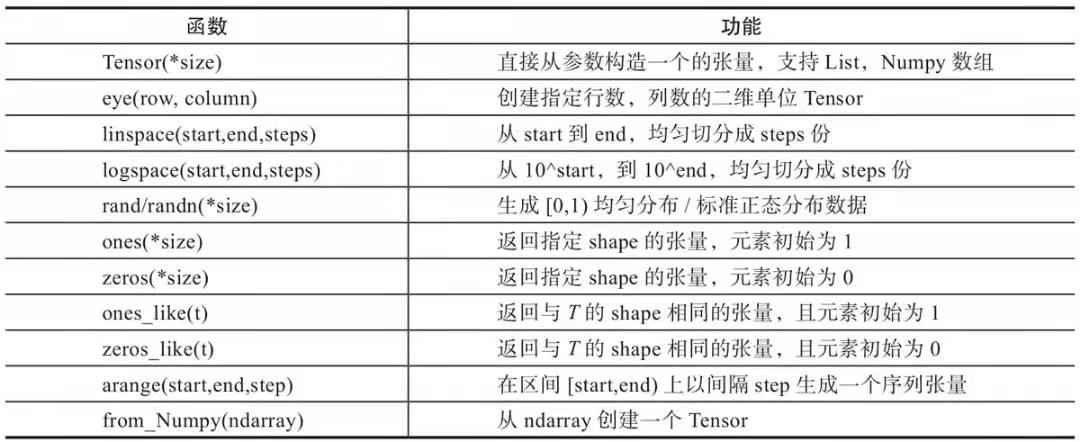（torch.Tensor与torch.tensor区别： 1.当传入数据时，torch.Tensor使用全局默认dtype（FloatTensor），而torch.tensor是从数据中推断数据类型； 2.torch.tensor(1)返回一个固定值1，而torch.Tensor(1)返回一个大小为1的张量，它是随机初始化的值）
2.修改Tensor的形状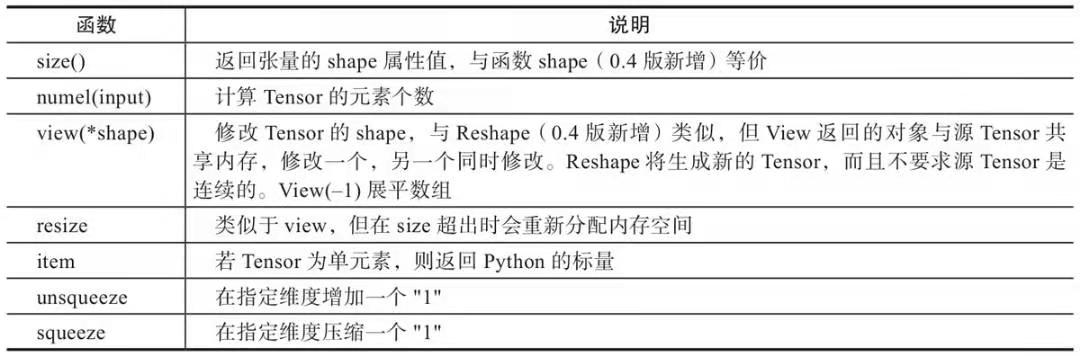3.常用选择操作函数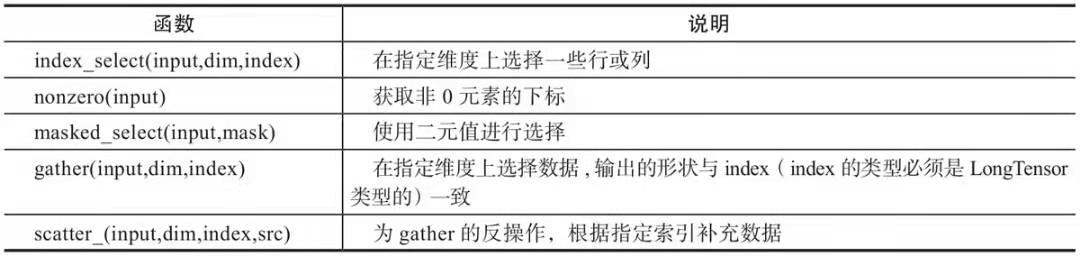4.常见逐元素操作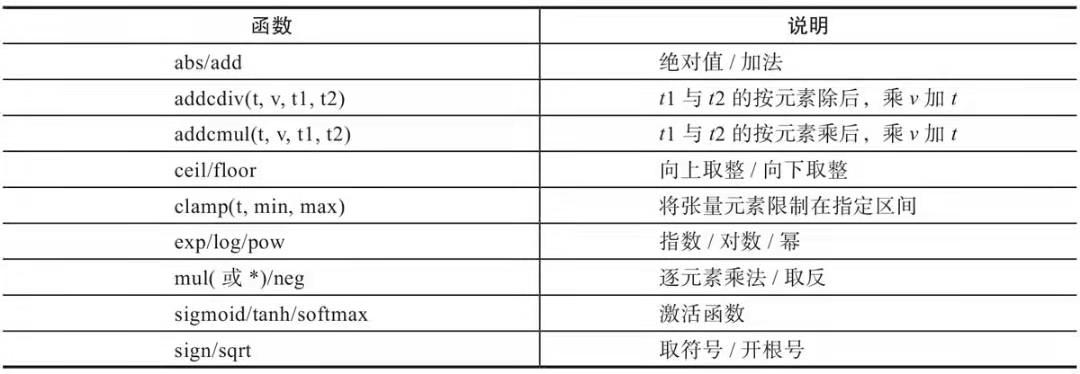5.常见归并操作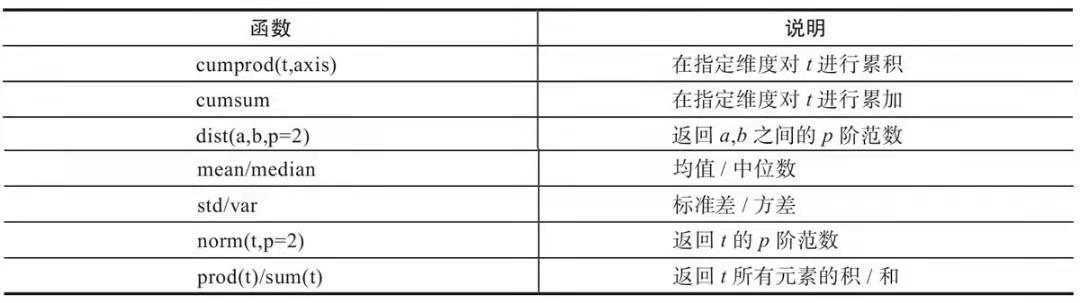6.常见比较操作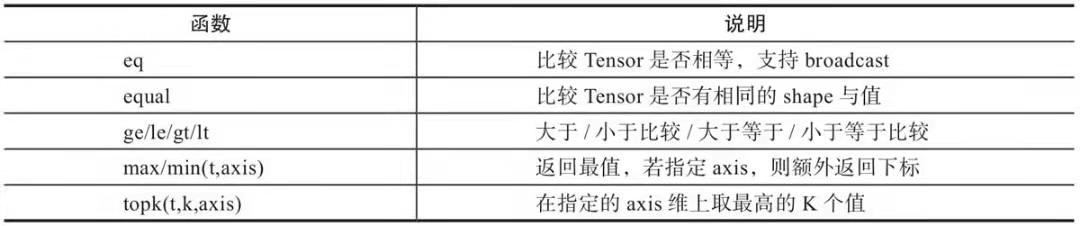7.常用矩阵函数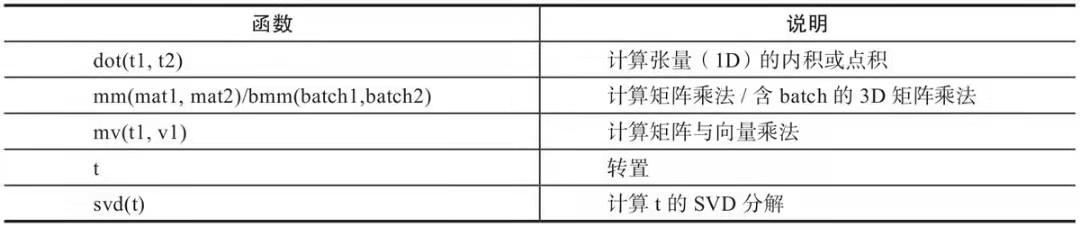8.PyTorch与Numpy函数对照表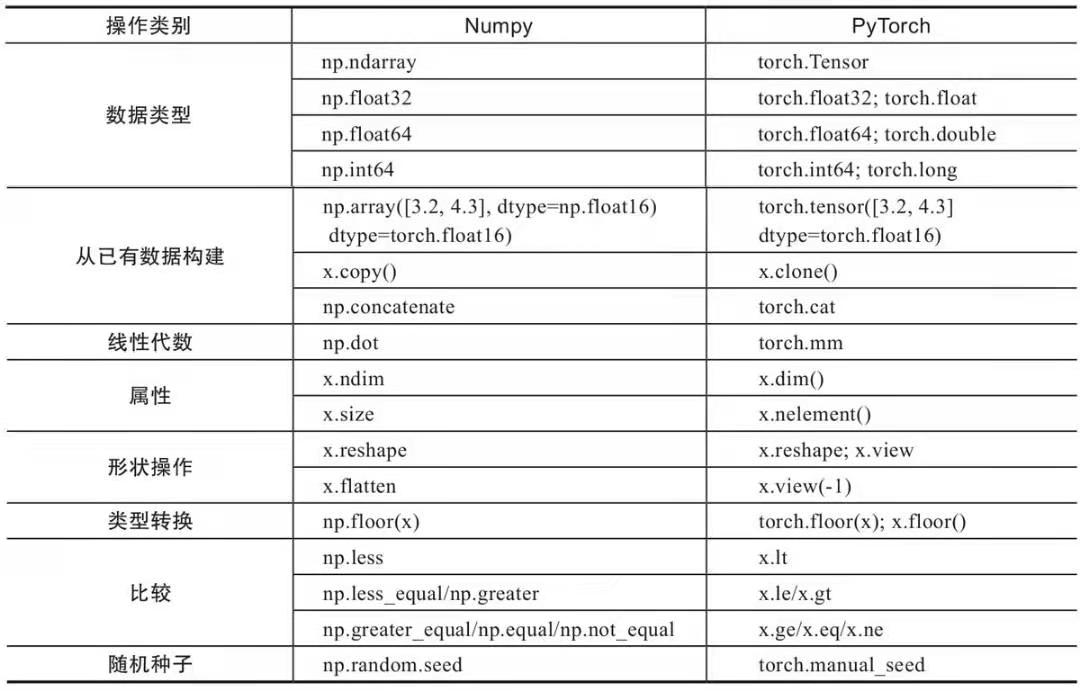注：本文根据微信读书《Python深度学习：基于PyTorch》整理。 链接: Python深度学习：基于PyTorch.
展开全文python numpy
• 一、PyTorch 是什么 PyTorch是一个基于Python的科学计算包，相比numpy能使用GPU来加快计算。 二、基本语法 2.1 张量（Tensors） 张量张量类似于numpy的ndarrays，不同之处在于张量可以使用GPU来加快计算。 构建...
一、PyTorch 是什么
PyTorch是一个基于Python的科学计算包，相比numpy能使用GPU来加快计算。
二、基本语法
介绍pytorch基本知识，对pytorch有基本的了解，构建基本的神经网络。主要参考pytorch官方教程。
2.1 张量（Tensors）
创建Tensor
函数说明torch.tensor(data )赋值torch.arange(start,end,step=1,out=None)torch.zeros(*sizes )全部生成为0torch.clone()torch.ones(*sizes )全部生成为1torch.eye(n,m=None,out=None)返回一个2维张量，对角线位置全为1，其他位置全0torch.from_numpy(ndarray)tensor和numpy的转换torch.linspace(start, end, steps=100, out=None)返回一个1维张量，包含在区间start和end上均匀间隔的step个点。torch.logspace(start,end,steps=100.out=None)设置的区间为常用对数，输出的值为其对应的真数
创建随机Tensor
函数说明torch.rand(*sizes, out=None)从区间[0, 1)的均匀分布中抽取的一组随机数torch.randperm(n,out=None)返回一个从0到n-1的随机整数排列torch.randn(*sizes, out=None)从标准正态分布（均值为0，方差为1，即高斯白噪声）中抽取的一组随机数torch.normal(means, std, out=None)指定均值means和标准差std的离散正态分布中抽取的一组随机数。uniform(from,to)均匀分布
常用Tensor操作
函数说明view(*shape)调整tensor的形状,与源tensor共享内存torch.squeeze(input, dim=None, out=None)删除尺寸1的输入的所有尺寸的张量torch.unsqueeze(input, dim, out=None)插入在指定位置的尺寸标注尺寸的新张量。resize修改tensor的尺寸None添加一个轴a > 1返回一个bool矩阵gather(input, dim, index)根据index，在dim维度上选取数据，输出的size与index一样index_select(input, dim, index)在指定维度dim上选取，比如选取某些行、某些列masked_select(input, mask)例子如上，a[a>0]，使用ByteTensor进行选取non_zero(input)非0元素的下标
数值计算
函数说明abs/sqrt/div/exp/fmod/log/pow…绝对值/平方根/除法/指数/求余/求幂…cos/sin/asin/atan2/cosh…相关三角函数ceil/round/floor/trunc上取整/四舍五入/下取整/只保留整数部分clamp(input, min, max)超过min和max部分截断sigmod/tanh…激活函数torch.lerp(star, end, weight)返回结果是out= star t+ (end-start) * weighttorch.equal(torch.Tensor(a), torch.Tensor(b))两个张量进行比较，如果相等返回true，否则返回falsetorch.max(input)返回输入元素的最大值mean/sum/median/mode均值/和/中位数/众数norm/dist范数/距离std/var标准差/方差cumsum/cumprod累加/累乘gt/lt/ge/le/eq/ne大于/小于/大于等于/小于等于/等于/不等topk最大的k个数sort排序max/min比较两个tensor最大最小值
线性代数计算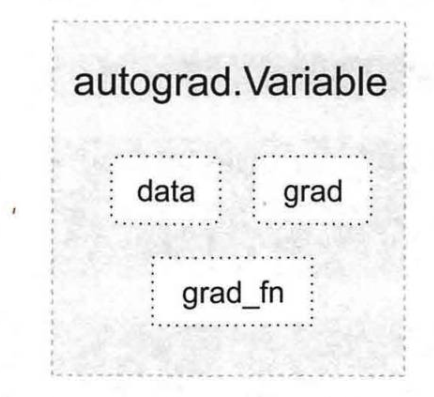Variable 支持大部分tensor支持的函数，但其不支持部分inplace函数，因为这些函数会修改tensor自身，而在反向传播中，variable需要缓存原来的tensor来计算梯度。 如果想要计算各个Variable的梯度，只需调用根节点variable的backward方法，autograd会自动沿着计算图反向传播，计算每一个叶子节点的梯度。
2.3 构建神经网络
三、常用函数
索引，切片，连接，换位
|.torch.transpose(input, dim0, dim1)|转置| |.torch.masked_select(input, mask, out=None) |根据二进制掩码对输入进行索引，这是一个新的索引| |torch.chunk(tensor, chunks, dim=0) |将张量拆分为特定数量的“块”| |torch.narrow(input, dimension, start, length)| |torch.stack(seq, dim=0, out=None) |拼接|
参考文献： pytorch官网 深度学习框架pytorch：入门与实践 PyTorch 深度学习:60分钟快速入门 torch—pytorch常用函数 Pytorch常用函数操作总结
展开全文• 矩阵乘法、克罗内克、哈达马则是矩阵论中的概念，强调的是更为一般性的n维向量的运算规则，矩阵内操作向量在内积空间中的矩阵乘法。 矩阵乘法是使用最多的运算，比如在 torc h中 * 、@、mm函数。点乘可以视...
• PyTorch sepconv-slomo通过自适应可分卷实现视频帧插值的实现。这是使用PyTorch通过自适应可分卷积实现视频帧插值的参考实现。 给定两个帧，它将以可分离的方式利用自适应卷积对中间帧进行插值。 如果您要...
• ## Pytorch API备忘

千次阅读 2018-09-11 14:49:05
之前一直用tensorflow和keras，最近在看一些CV领域的paper，发现相关的开源代码很多是pytorch实现的，于是打算学下pytorch。 以下内容主要来于《深度学习入门之Pytorch》这本书。 Pytorch基础 Tensor张量 ...
• 强烈安利，拿走不谢，顺手留个关注和个赞，嘿嘿嘿 PyTorch我就不多说了吧，我搞CV平时也就用PyTorch和TensorFlow，不过最近更喜欢用PyTorch 了，因为TensorFlow的API真的搞的我头疼，不过因为我有时候用C++写算法...Python 自然语言处理 计算机视觉
• pytorch调用的函数是F.normalize。一般池化后会有一层l2norm层。 # l2norm 的实现如下 x = torch.tensor([[1, 2, 3], [4, 5, 6], [7, 8, 9]]) norm = x.pow(p).sum(1, keepdim=True).pow(1./ p) # l2norm p=2 print...python 深度学习
• pytorch10 pytorch常见运算详解pytorch常见运算详解矩阵与标量哈达玛矩阵乘法幂与开方对数运算近似值运算剪裁运算 矩阵与标量 这个是矩阵（张量）每一个元素与标量进行操作。 import torch a = torch.tensor([1,2]...
• 转自：... bool = torch.is_tensor(x)：判断x是否为pytorch张量； bool = torch.is_storage(x)：判断x是否为pytorch对象； N = torch.numel(x)：输出pytorch张量x的元素数； x = torch.ey...
• 连乘 1.应用 import torch import torch.nn as nn a = torch.randn(1, 2, 3) torch.numel(a) # 1*2*3 = 6 a = torch.zeros(4,4) torch.numel(a) # 4*4 = 16 API torch.numel(input) → int 参数 描述 ...python
• ## Pytorch 叉乘

千次阅读 2018-10-03 14:58:11
使用Pytorch时遇到想要将矩阵进行自身叉乘的情况，例如输入数据size为[BS, 3]，那么需要先将输入数据转换为[BS, 3, 1]和[BS, 1, 3]，这时叉乘结果size为[BS, 3, 3] 下面为二维矩阵的pytorch代码及结果： import ...
• #返回输入参数的求结果 import torch a = torch . randn ( 2 , 3 ) print ( a ) b = torch . randn ( 3 ) print ( b ) c = torch . mv ( a , b ) print ( c ) tensor([[ 1.5491, -0.0762, ...
• t.dot()/t.cross() # 内/外 t.inverse() # 求逆矩阵 t.svd() # 奇异值分解 # tensor与numpy t.from_numpy() # 这种情况，numpy与tensor共享内存 t.Tensor(numpy) # 这种方式，如果numpy类型不是Float32会新建 ...
• pytorch杂记forward方法Conv2d方法GRU方法 forward方法 nn.Module中的forward方法没有实际内容，用于被子类的 forward() 方法覆盖，且 forward 方法在__call__方法中被调用： forward方法的具体流程： 以一个Module...
• 1.2 inner product, 向量内 1.3 torch.mul(a, b) 元素对应相乘 （element wise） 1.4 将numpu转为tensor: torch.from_numpy()，如果要使其求导，转化为torch.float型   1.5 RuntimeError: grad can ...
• 点积注意力 多层感知机注意力 seq2seq模型 transformer cnn与rnn的区别 多头注意力基于位置的前馈网络 add and norm 位置编码 优化与估计 局部最小值，鞍点，梯度消失 凸性、jensen不等式 梯度下降 一维梯度下降、...
• ## pytorch求导

千次阅读 2020-03-27 10:13:18
前言：构建深度学习模型的基本流程...由损失函数求导的过程，称为“反向传播”，求导是件辛苦事儿，所以自动求导基本上是各种深度学习框架的基本功能和最重要的功能之一，PyTorch也不例外，后面有时间会写一下tens...人工智能 python
• 文章目录**资源汇总1 PyTorch Tutorials: [入门-1 60分钟闪电战](https://pytorch.org/tutorials/beginner/deep_learning_60min_blitz.html)1.1 [什么是PyTorch]...深度学习 神经网络
• 目的：熟悉Pytorch框架, 搭建简易滑神经网络 结构： 代码： Imports import torch import torch.nn as nn import torchvision.datasets as dsets from skimage import transform import torchvision....
• 在这篇文章中：一、神经网络（Neural Network）二、卷积神经网络（Convolutional Neural Network）1、局部感知2、参数共享3、Pooling4、卷积神经网络的构建三、卷积神经网络CNN的PyTorch实现四、CNN常见模型之ResNet...python 神经网络 深度学习
• 神经网络）工作方式的大体概述。 CNN 工作 【1】卷积层、 【2】非线性层、 【3】池化（下采样（downsampling））层 【4】全连接层， 最终得到输出。输出可以是最好地描述了图像内容的一个单独分类或一组分类的概率...卷积 神经网络 网络 深度学习 计算机视觉
• 基于MPI的点积注意力分布的PyTorch实施 概述 该软件包为大多数Transformer模型和非本地块中的点积注意力运算符所涉及的主要线性运算提供了多GPU，运算符级别的分布式实现。 ， 和。 此实现基于单批时间相关线性......